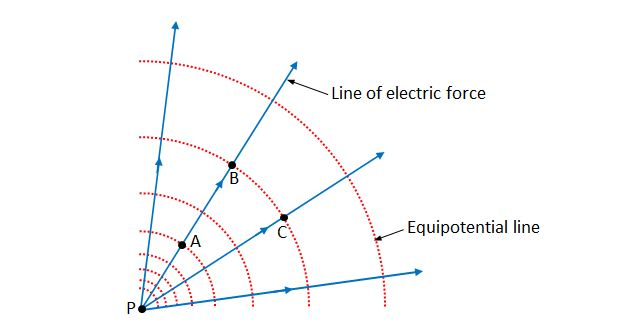# Electric field linesThe above diagram shows the lines of electric force and equipotential lines on a particular plane. Which of the following statements is correct?

a) The electric potential at point A is higher than that at point B.
b) The electric field strength at point A is the same as that at point B.
c) The work done by the electric force when an electrically charged particle is moved from point B to C along the equipotential line is zero.

×